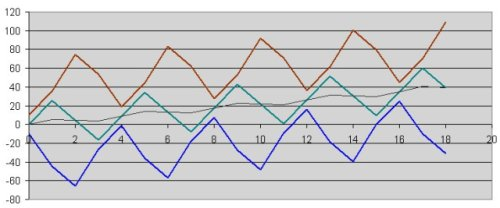Flow Resistance of double spheres in viscous fluid

The propulsion of the 3 sphere system relies on the different flow resistance the spheres have when they move in different directions. The sphere themselves are just round and have the same flow resistance in each direction, but they come together to form a close group before moving into the heading direction while they return separately. So using the slipstream of a pair, an overall propulsion becomes effective.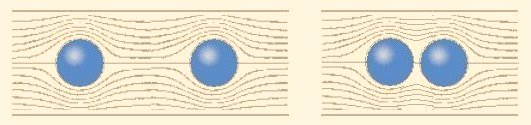For the analysis the slipstream will be measured at two spheres. For the definition a displacement d is introduced to describe the distance of the spheres. The displacement is the difference of location of the two spheres. With the displacement 0 the two spheres are at the same location and are just one sphere (with twice the weight). With the displacement d1 (one diameter) the spheres are in contact. Higher values of the displacement are possible up to the value "infinity" where the spheres are so far from each other that there is no slipstream and the resistance is exactly twice the resistance of one sphere.

This definition helps to construct a diagram figure 9 with known values at the borders at 0 and infinity. There the values for the resistance (relative to the resistance of one sphere) are 1 and 2.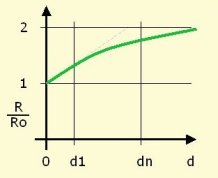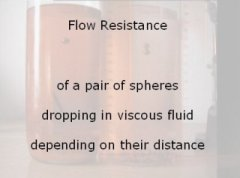Figure 9 shows the principle form of the function, particular values for the resistance were measured with 7mm steel spheres dropping in viscous liquid. Dropping a single sphere gives the reference value.

Two of the spheres can be attached to an iron wire equipped with a magnet. Dipping the wire into the liquid and pushing it aside with a small hammer releases the two spheres attached to each other to measure the dropping speed and the resistance as the reciprocal value.

Releasing two spheres with a certain displacement between them proved difficult enough to construct a small tool made of two iron wires with two magnets.

The resulting curve measured in 5 points is shown in fig 11, together with an approximation term for the relevant section.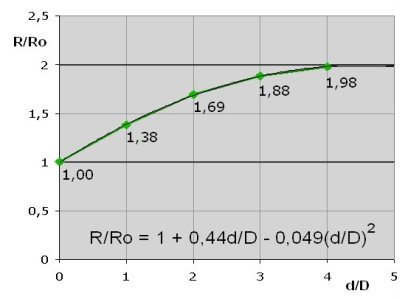Description of the movement

Calculation Example

For a simple model of the functionality the cycle of the movement is divided in four phases (see fig 12):

left sphere moving out,
right sphere moving out,
left sphere moving in.
right sphere moving in. .

For each of the four phases the system is divided into subsystems in such a way, that the spheres in a subsystem do not move against each other.

With that Model there are three types of subsystems:

A subsystem out of one sphere with the relative flow resistance 1.
A subsystem out of two spheres attached to each other with the relative flow resistance 1,38.
A subsystem out of two spheres with the displacement of 3 diameters with the relative flow resistance 1,88.

When these subsystems move, their velocity is reciprocal to their flow resistance.For the left sphere moving out, the left sphere moves for 0.58 of the elementary distance to the left while the other two spheres move 0.42 of the elementary distance to the right. In the next phase when the right sphere moves out, the right sphere moves for 0.65 to the right and the other two move 0.35 to the left.

For the sphere in the middle this means 0.42 - 0.35 - 0.35 + 0.42 = 0.14 elementary distances (of 60mm) per cycle. For the outer spheres the distance is calculated in the picture with the same results. This is a movement of 8.75mm per cycle.

(For the real system with the sinusoidal movement the value was measured with 4,8mm. The deviation shall be further investigated. For a first calculation the result is quite in a good range. )

For this description a movement can be calculated that results in a position of the spheres according to fig13. The curves look similar to the measured curves.

-> next page